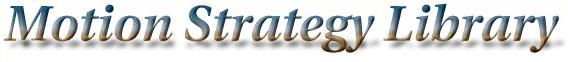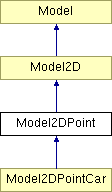Main Page   Namespace List   Class Hierarchy   Compound List   File List   Compound Members   File Members

# Model2DPoint Class Reference

A point robot in a 2D world. More...

`#include <model2d.h>`

Inheritance diagram for Model2DPoint:List of all members.

## Public Methods

Model2DPoint (string path)
virtual ~Model2DPoint ()
virtual MSLVector Integrate (const MSLVector &x, const MSLVector &u, const double &h)
Perform integration from state x, using input u, over time step h. More...

virtual MSLVector StateTransitionEquation (const MSLVector &x, const MSLVector &u)
The state transition equation, or equations of motion, xdot=f(x,u). More...

virtual double Metric (const MSLVector &x1, const MSLVector &x2)
A distance metric, which is Euclidean in the base class. More...

## Detailed Description

A point robot in a 2D world.

## Constructor & Destructor Documentation

 Model2DPoint::Model2DPoint ( string path )

 virtual Model2DPoint::~Model2DPoint ( ) ` [inline, virtual]`

## Member Function Documentation

 MSLVector Model2DPoint::Integrate ( const MSLVector & x, const MSLVector & u, const double & h ) ` [virtual]`
 Perform integration from state x, using input u, over time step h. Implements Model. Reimplemented in Model2DPointCar.

 double Model2DPoint::Metric ( const MSLVector & x1, const MSLVector & x2 ) ` [virtual]`
 A distance metric, which is Euclidean in the base class. Reimplemented from Model. Reimplemented in Model2DPointCar.

 MSLVector Model2DPoint::StateTransitionEquation ( const MSLVector & x, const MSLVector & u ) ` [virtual]`
 The state transition equation, or equations of motion, xdot=f(x,u). Implements Model. Reimplemented in Model2DPointCar.

The documentation for this class was generated from the following files: Motion Strategy Library

Web page maintained by Steve LaValle